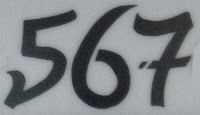## Wednesday, November 9, 2011

### 567

567 = 34 x 7.

567 is the smallest possible sum of primes that are formed using all 10 digits: 567 = 401 + 89 + 67 + 5 + 3 + 2.

567 is the smallest number that together with its square (5672 = 321489) uses the digits 1 to 9 exactly once.

567 is 4232 in base 5 and 2343 in base 6. It is 700 in base 9.

567 is a divisor of 266 - 1.The EMD 567 is a line a diesel engines produced by General Motors from 1938 until 1966 for locomotives.